Define Me!
Table of Values
The Number Plane
Linear Equations
Miscellaneous
100

Definition of the y-intercept of a line.

The y-intercept of a line is the value at which the line crosses the y-axis.

100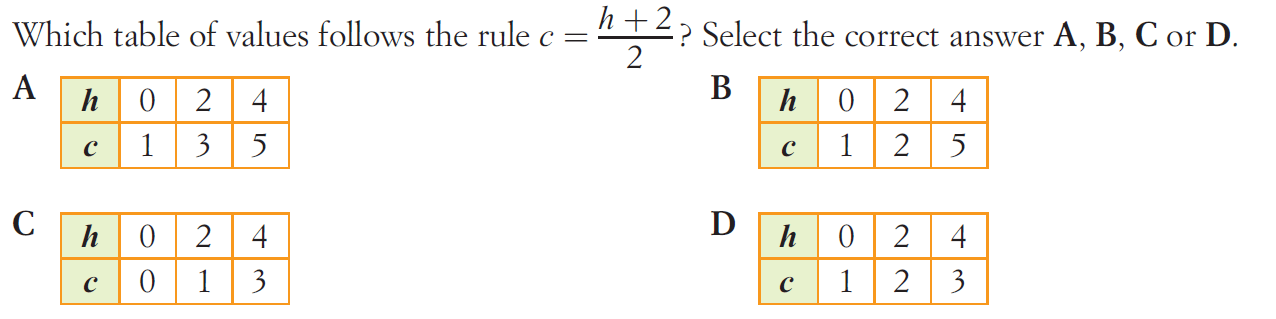D

100

What is another name for the number plane? Bonus 100 points, if you remember who it was named after.

The Cartesian Plane

Bonus: Rene Descartes

100

A linear equation is an equation or formula whose graph on a number plane is what?

A straight line

100

What two activities did we do in our very first lesson together? Bonus 100, if you can remember the day.

1. Race to 3pm (board game)

Bonus: Friday

200

In a linear equation, what is the variable term?

The term with the x.

200

Complete the table of values.200

The number plane is divided evenly into four regions called ______________.

200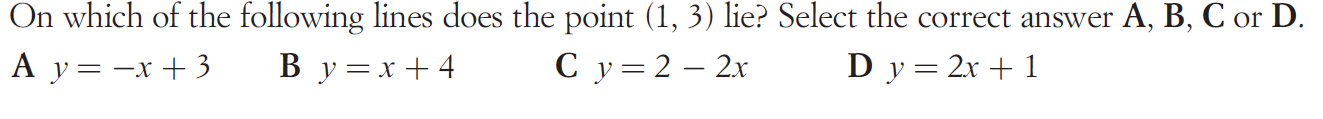D

200

In the PowerPoint slides, what picture do I use to represent our lesson goals?

A target.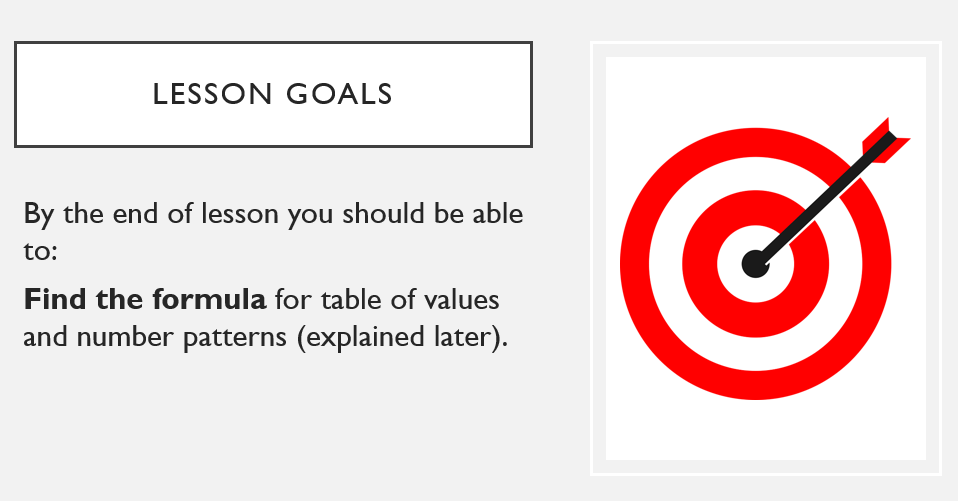300

In a linear equation, what is the constant term?

The number without the x.

300C

300

In any ordered pair, the first number is called the a)________________ and the second number is called the b)____________________.

a) x-coordinate

b) y-coordinate

300

Find the equation of the line.y = 1/2x + 2

300

What is the brand of the teacher's laptop?

ASUS

400

In a linear equation, what is the number in front of the x called? What does it tell us about the line?

The coefficient - gives us the direction of the line.

400

Find the formula for the table, then complete the last two columns.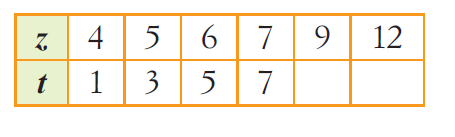t = 2z -7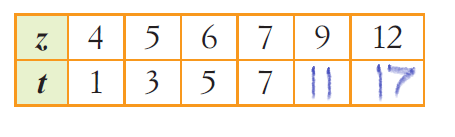400

Points that lie on the same straight line are said to be ______________.

Collinear

400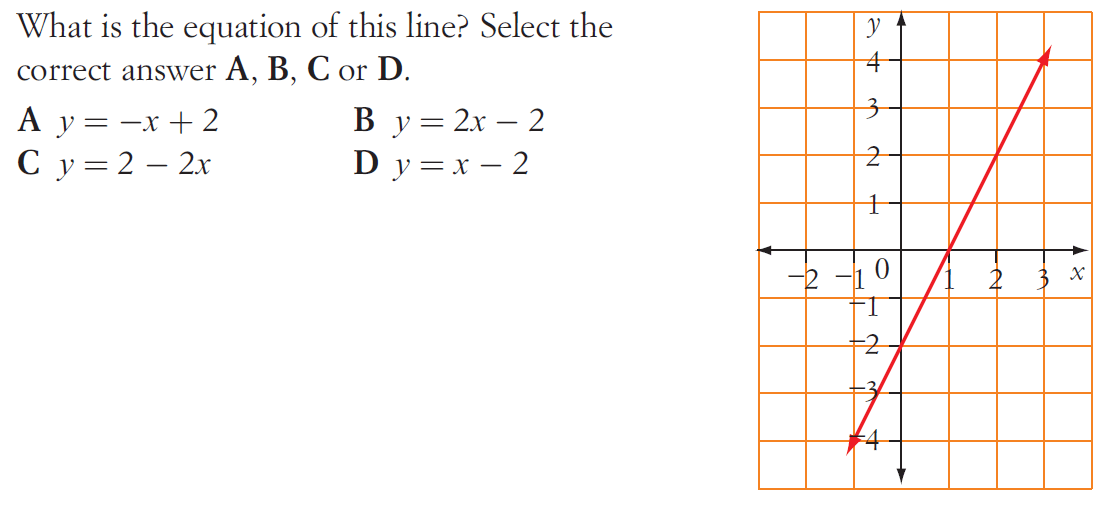B

400

What is the colour of the teacher's bottle and pencil case?

Bottle: yellow

Pencil case: pink

Click to zoom